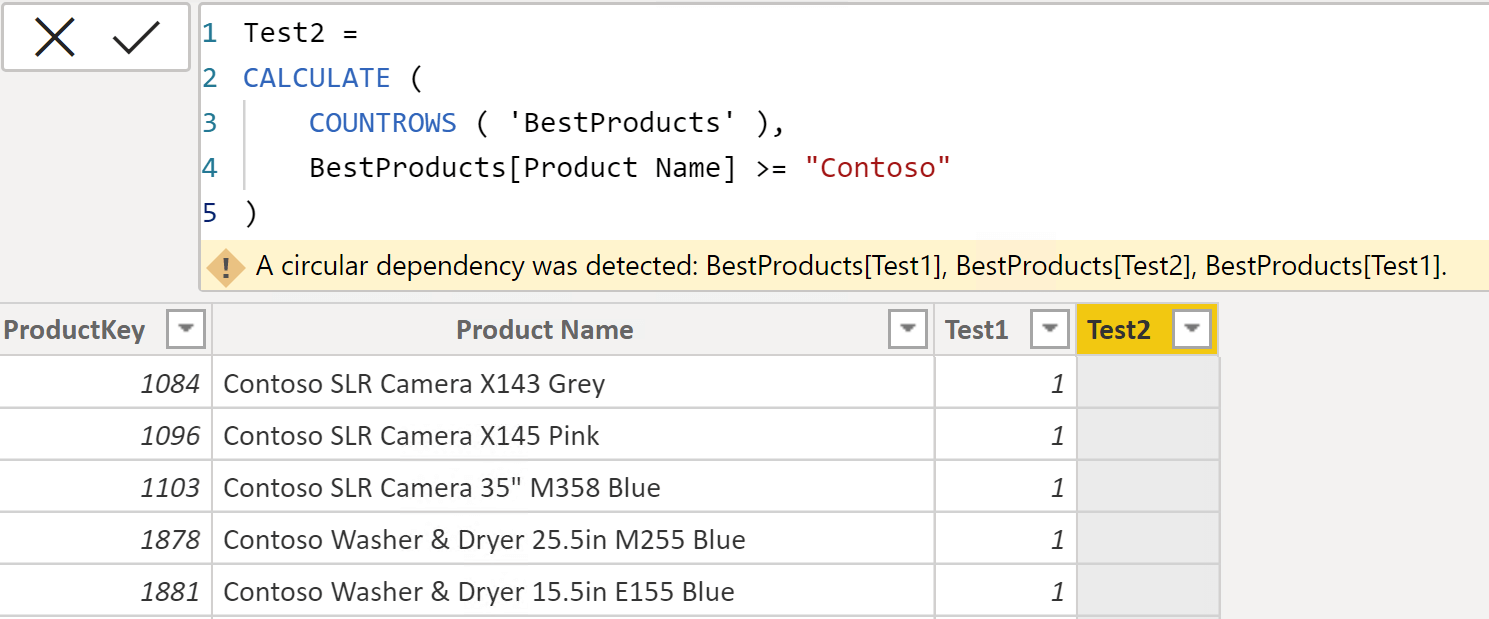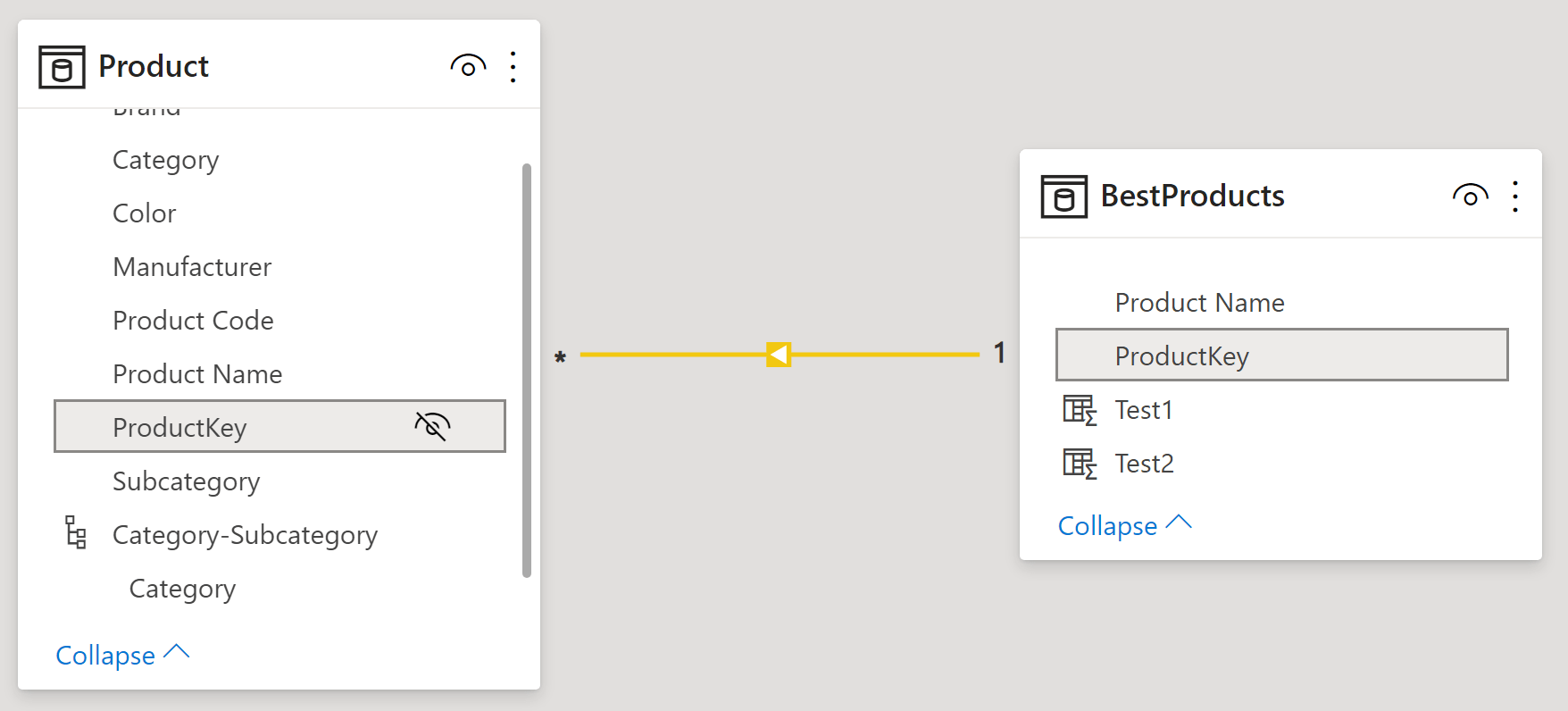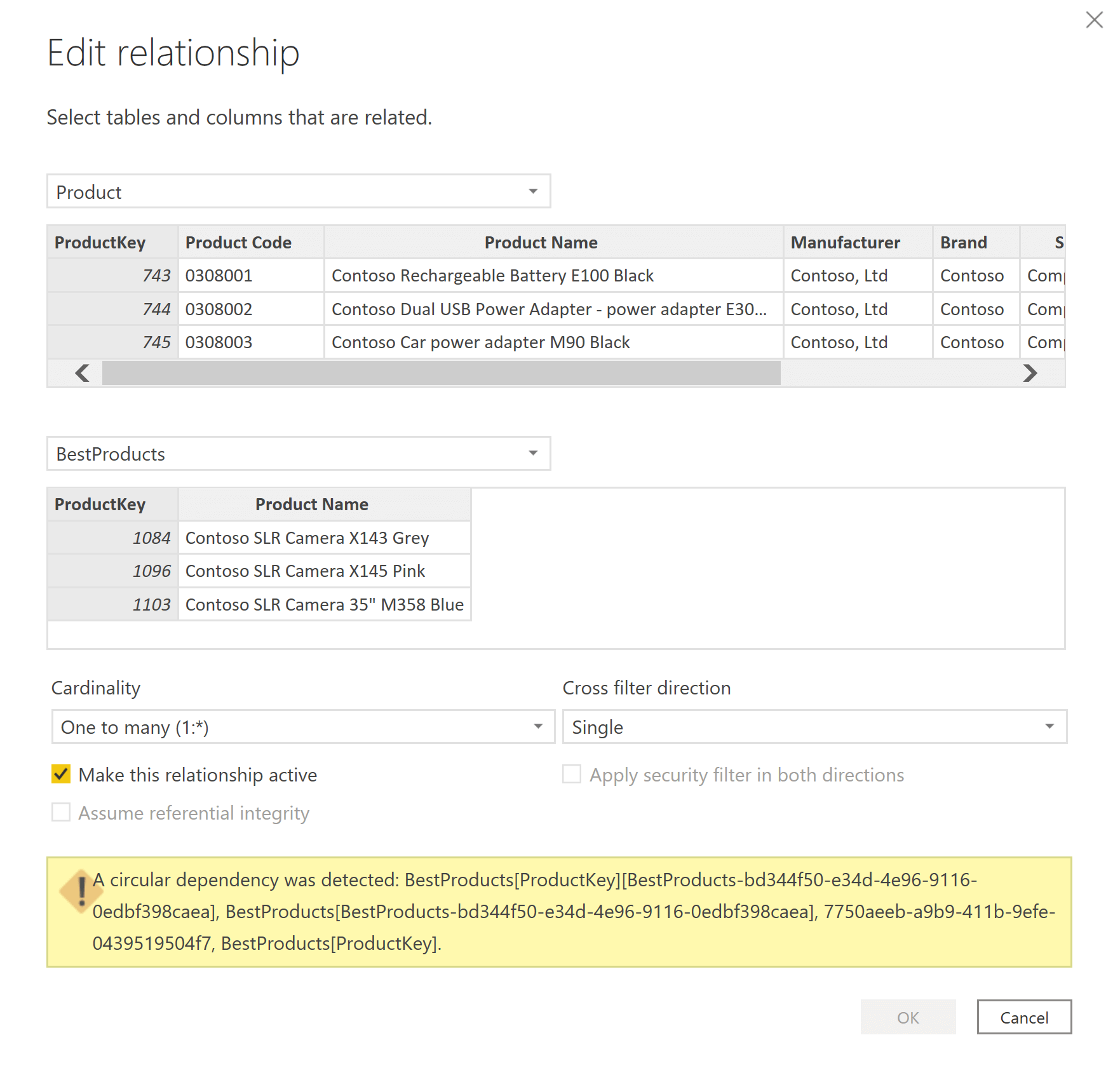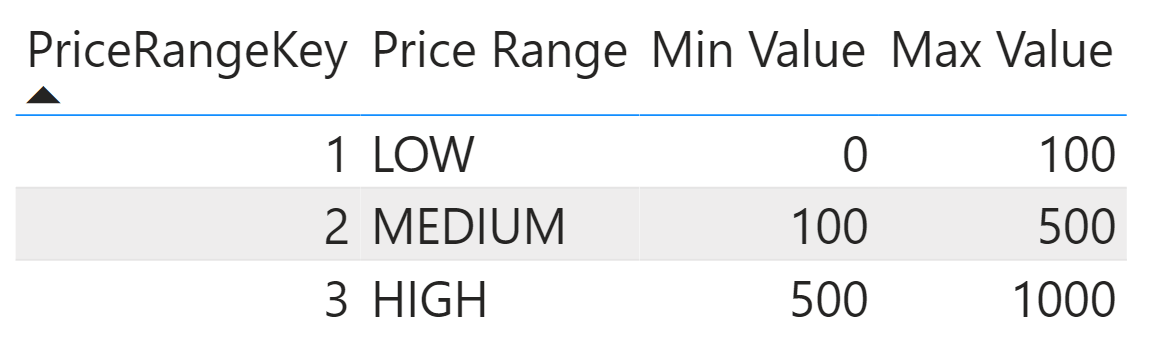Calculated objects often reference each other. For example, you create three calculated columns in Sales to compute Line Amount, Line Cost, and Line Margin:

```Line Amount = Sales[Quantity] * Sales[Net Price]
Line Cost = Sales[Quantity] * Sales[Unit Cost]
Line Margin = Sales[Line Amount] - Sales[Line Cost]
```

Line Amount and Line Cost are calculated based on columns already present in the table. Line Margin requires the values of both Line Amount and Line Cost in order to produce a result. Therefore, DAX knows that it must compute Line Amount and Line Cost before computing Line Margin. With three columns, it is somewhat easy to track the dependencies. In a real model with many columns, the dependencies are much more complex to follow. That said, the DAX engine keeps track of which entity depends on which. It uses a dependency diagram to build an execution plan where each entity is computed after all the objects which the original entity depends on. The good news is that you do not have to worry about any of this. The engine does a splendid job of managing the complexity of dependency graphs all by itself.

The dependency diagram is used when data is refreshed. If you update one column, all the columns that depend on the original column are recomputed. A very practical way of understanding dependencies is to think that if A depends on B, it means that if B changes its value, then A might change its value too. Therefore, when B changes, A needs to be recalculated.

In some scenarios, the DAX engine cannot build the dependency diagram. If two objects depend on each other, the engine does not know which one to compute first. If A depends on B and – at the same time – B depends on A, then it is impossible to compute A nor B. As soon as you compute A, this triggers the refresh of B. So you start refreshing B, wich in turn triggers the refresh of A. You are stuck in an infinite loop with no hope of ever getting the job done.

This situation, an infinite loop in the query plan, triggers the infamous circular dependency error. The only way to get rid of the problem is to avoid any circular dependencies. A model containing circular dependencies cannot be processed until the developer removes them entirely from the dependency chart. It is as simple as that.

Besides, circular dependency is such an intuitive topic that developers very seldom write circular code. It is actually challenging to even conceptualize objects that depend on one another in a circular way. If you have ever worked with recursive languages, you know how counterintuitive it is to design your code using recursion.

Now that we have outlined what circular dependency is, it is time to understand why Power BI (or Tabular) sometimes refuses to accept the creation of a column or of a relationship – stating that if you create that column (or relationship) you introduce a circular dependency error. There is a good chance that you have landed on this article by searching exactly for the error this scenario would return; or you have arrived here after reading the Avoiding circular dependency errors in DAX article.

When Power BI complains about a circular dependency, you can be sure that it is right. The dependency tracking algorithm is rock solid. What falls under the responsibility of the developer (that is, you!) is to locate where the circular situation is happening. This last step requires some work because circular dynamics are either very evident or incredibly well hidden. If not evident (it seldom is), then finding the problem requires for you to understand certain intricacies in the dependencies. We are not going to talk about the simplest scenarios where the code that was written is clearly wrong. Instead, we describe the most common hidden causes for circular dependencies.

You can obtain a circular dependency in two main scenarios:

• When creating a calculated column that looks perfectly legitimate and that uses CALCULATE;
• When trying to create a relationship between two tables, one of which is a calculated table, or when the relationship is based on a calculated column.

Albeit raising the same error, the two scenarios are very different. Circular dependencies occur with calculated columns when you obtain a dependency list that is much wider than you would expect because of context transition. On the other hand, relationships might introduce circularity because of the very special way in which Tabular handles invalid relationships: by adding a blank row.

Out of the two, circular dependency in calculated columns is the less frequent scenario. Despite being the rarest case, it is also simpler to understand. Hence, we start with circular dependencies in calculated columns.

## Circular dependencies in calculated columns

Be warned that the examples we are using in the remaining part of the article are educational only. We are not meaning to show a practical scenario. Instead, we artificially create useless tables and columns for the sole purpose of understanding circular dependency.

In order to demonstrate circular dependency, we need a table with special characteristics: it must be unrelated to the model, but still capable of being related. A copy of the Product table would be a perfect fit. To save time, we build a table that will be useful later to describe a circular dependency in relationships. The new calculated table used for this demo is BestProducts. It contains the top five percent of the products ordered by their sales in 2008:

```BestProducts =
CALCULATETABLE (
VAR MinSale = PERCENTILEX.INC ( 'Product', [Sales Amount], 0.95 )
VAR Result =
SELECTCOLUMNS (
FILTER (
ALL ( 'Product' ),
[Sales Amount] >= MinSale
),
"ProductKey", 'Product'[ProductKey],
"Product Name", 'Product'[Product Name]
)
RETURN Result,
'Date'[Calendar Year] = "CY 2008"
)
```

After we create the BestProducts table, we build two identical calculated columns in it:

```Test1 = CALCULATE ( COUNTROWS ( 'BestProducts' ), BestProducts[Product Name] >= "Contoso" )
Test2 = CALCULATE ( COUNTROWS ( 'BestProducts' ), BestProducts[Product Name] >= "Contoso" )
```

The first calculated column Test1 is created without issues. To our surprise, the second calculated column Test2 – despite being identical to the first one – raises the circular dependency error.The reason for the circular dependency is that the dependency list of Test1 (and of Test2) is wider than expected. Indeed, by quickly looking at the code, one would say that both Test1 and Test2 depend on the value of Product Name, because Product Name is the only column used in the filter expression. By drawing that conclusion, the developer would be missing a very important point: CALCULATE is being used in the expression, and the formula is evaluated in the row context of the calculated column.

When CALCULATE runs inside a row context, it performs a context transition. Context transition places a filter on all the columns currently being iterated to match the currently iterated value. In other words, Test1 in the first row would be executed this way:

```CALCULATE (
CALCULATE (
COUNTROWS ( 'BestProducts' ),
BestProducts[Product Name] >= "Contoso"
),
BestProducts[ProductKey] = 1084,
BestProducts[Product Name] = "Contoso SLR Camera X143 Grey"
)
```

The outer CALCULATE is the result of context transition, which happens before explicit filters are applied; this is why we added context transition as an outer CALCULATE, instead of placing all the filters side-by-side.

If you read the expanded code, it is now evident that the formula depends not only on Product Name, but also on ProductKey. Besides, in this example we have only two columns. If we used Product, we would have needed all the columns of Product specified in the explicit filters, resulting in a much longer dependency list.

That said, creating Test1 works fine. The column that causes us trouble is Test2. Indeed, what would happen if you were able to create Test2 without getting the circular dependency error? The BestProduct table would contain two additional columns, and the code would become:

```Test1 =
CALCULATE (
CALCULATE (
COUNTROWS ( 'BestProducts' ),
BestProducts[Product Name] >= "Contoso"
),
BestProducts[ProductKey] = 1084,
BestProducts[Product Name] = "Contoso SLR Camera X143 Grey",
BestProducts[Test2] = 1
)

Test2 =
CALCULATE (
CALCULATE (
COUNTROWS ( 'BestProducts' ),
BestProducts[Product Name] >= "Contoso"
),
BestProducts[ProductKey] = 1084,
BestProducts[Product Name] = "Contoso SLR Camera X143 Grey",
BestProducts[Test1] = 1
)
```

As you see, the code of Test1 references Test2; at the same time, the code of Test2 references Test1. DAX thus complains about a circular dependency, and it proactively refuses to accept the creation of Test2.

The same reasoning explains why you can create one calculated column with CALCULATE, but you cannot create the second one. In the absence of Test2, Test1 does not reference columns other than the ones already present in the table. It is the creation of Test2 that changes the code of Test1. Indeed, DAX is very wise in preventing us from introducing any circular dependency.

There are two possible solutions to this kind of circular dependency: changing the dependency list by using DAX code, or relying on one specific DAX optimization. The former is safer, though a bit more verbose; the latter is simpler to implement, albeit less safe.

The first option is to change the behavior of the context transition by introducing ALLEXCEPT. When using ALLEXCEPT you can remove columns from the context transition, thus keeping in the dependency list only the required columns. If you author the code of both columns this way, you can create both calculated columns and yet avoid the circular dependency problem:

```Test2 =
CALCULATE (
COUNTROWS ( 'BestProducts' ),
BestProducts[Product Name] >= "Contoso",
ALLEXCEPT ( BestProducts, BestProducts[ProductKey] )
)
```

By using ALLEXCEPT, we have removed all filters except for the one on ProductKey. Indeed, because ProductKey is the table’s primary key, the context transition can be completed by applying a filter only on that column.

By using the previous code based on ALLEXCEPT for both Test1 and Test2, the context transition produces the following formulas for the two columns:

```Test1 =
CALCULATE (
CALCULATE (
COUNTROWS ( 'BestProducts' ),
BestProducts[Product Name] >= "Contoso"
),
BestProducts[ProductKey] = 1084
)

Test2 =
CALCULATE (
CALCULATE (
COUNTROWS ( 'BestProducts' ),
BestProducts[Product Name] >= "Contoso"
),
BestProducts[ProductKey] = 1084
)
```

The circular dependency is no longer present; neither does Test1 depend on Test2, nor the other way around.

You can also obtain the same result if you create a relationship between Sales and BestProducts based on ProductKey. The presence of a relationship forces the DAX engine to verify that ProductKey is a column with unique values in BestProducts. If there is a unique column in the table, the context transition is optimized to rely on that column, without filtering all the other columns.

This latter consideration is the reason why most developers have been able to create multiple calculated columns in dimensions. Because a dimension is the target of a relationship, DAX takes advantage of the optimization and seldom shows any form of circular dependency.

Beware that this latter technique relies on an optimization that may be turned off for several reasons. Here are a couple of scenarios where this optimization is not activated:

The technique using ALLEXCEPT relies on the semantics of the DAX language. As such, it works in any scenario, regardless of any optimization.

IMPORTANT: We strongly suggest you learn and understand the implications of context transition in calculated columns, and use ALLEXCEPT in order to remove any filter apart from the primary key of the table. This way your code is more stable, and future-proof.

## Circular dependencies in relationships

There is another common scenario where circular dependency errors happen and are hard to spot: when we create relationships. In the previous section, we suggested creating a relationship between Sales and BestProducts to eliminate the wider dependency list. If you tried that, you have obtained the model below.Product is on the many-side and BestProducts is on the one-side of a regular many-to-one relationship. This relationship works fine, but if you reverse it (using BestProducts on the many-side and Product on the one-side) you obtain a circular dependency error again. To make sure we are not polluting the example, we removed the Test columns and just tried to build the relationship.Why can we create the relationship in one direction but not the other way around? The reason – this time – is not in the dependency list. Indeed, there is no DAX code involved at this point. The reason is that the mere presence of a relationship creates a dependency between tables.

If Product is on the one-side of a many-to-one relationship with BestProducts, then Product depends on BestProducts. Besides, because BestProducts is a calculated table based on Product, the opposite is also true: BestProducts depends on Product. What remains to discover is why the presence of a relationship creates a dependency.

The reason is the blank row. If you are not familiar with the concept of the blank row, you should read the article, Blank row in DAX. The blank row is generated in the target of a relationship if the source table contains values for the key not present in the target table. In other words, depending on the content of BestProducts, Product might or might not contain the blank row. Hence the dependency.

Imagine you refresh Product. DAX computes BestProducts again because BestProducts depends on Product. After having computed BestProducts, it triggers the refresh of Products to add the potential blank row. This last step, in turn, triggers the processing of BestProducts again. Another nasty infinite loop that the Tabular engine refuses to accept.

If you have read up to this point, you probably think: ok, it is true that Product depends on BestProduct, but this dependency is somewhat weaker than a regular dependency. Indeed, the only detail in Product that depends on BestProducts is the presence of the blank row. Alas, the presence of the blank row affects BestProducts: BestProducts may or may not contain the blank row depending on whether it is present in Product. Therefore, as weak as the dependency may be, it still is a valid dependency.

If you want to create the relationship and yet avoid the error, you need to break one of the dependencies. Let us write down the two dependencies in this scenario:

• BestProducts depends on the content of the entire Product table, including the blank row.
• The blank row in Product depends on the content of BestProducts.

By slightly changing the first dependency, we might obtain a better scenario. What if we could build the model so that the requirements read this way:

• BestProducts depends on most of the content of the Product table: it never uses the blank row. Therefore, the content of BestProducts does not change depending on the presence of the blank row in Product.
• The blank row in Product depends on the content of BestProducts.

If the first requirement were stated that way, then the model would compute BestProduct first, then determine whether or not to add the blank row to Product and then… nothing more. There is no need to refresh BestProducts again because the presence of the blank row is irrelevant to BestProducts.

The engine can generate a dependency like the last one. If the code of BestProducts does not depend on the presence of the blank row , then the engine marks that dependency as “anything except the blank row”, and you can build the relationship.

To ensure that the code of BestProducts does not depend on the presence of the blank row, you need to use only functions that do not use the blank row. ALL is the major culprit in our code, but it is not the only one. Let us recall the code of BestProducts here:

```BestProducts =
CALCULATETABLE (
VAR MinSale = PERCENTILEX.INC ( 'Product', [Sales Amount], 0.95 )
VAR Result =
SELECTCOLUMNS (
FILTER (
ALL ( 'Product' ),
[Sales Amount] >= MinSale
),
"ProductKey", 'Product'[ProductKey],
"Product Name", 'Product'[Product Name]
)
RETURN Result,
'Date'[Calendar Year] = "CY 2008"
)
```

If you use ALLNOBLANKROW instead of ALL in the code of BestProducts, you go one step in the right direction. But there is another – very well hidden – ALL that still prevents the model from working properly.

Indeed, you might remember that CALCULATE filter arguments are tables, even though they are written as predicates. For example, the filter for CY 2008 is expanded by DAX this way:

```BestProducts =
CALCULATETABLE (
VAR MinSale = PERCENTILEX.INC ( 'Product', [Sales Amount], 0.95 )
VAR Result =
SELECTCOLUMNS (
FILTER (
ALL ( 'Product' ),
[Sales Amount] >= MinSale
),
"ProductKey", 'Product'[ProductKey],
"Product Name", 'Product'[Product Name]
)
RETURN Result,
FILTER (
ALL ( 'Date'[Calendar Year] ),
'Date'[Calendar Year] = "CY 2008"
)
```

If you want to build the relationship, you must replace both instances of ALL with ALLNOBLANKROW:

```BestProducts =
CALCULATETABLE (
VAR MinSale = PERCENTILEX.INC ( 'Product', [Sales Amount], 0.95 )
VAR Result =
SELECTCOLUMNS (
FILTER (
ALLNOBLANKROW ( 'Product' ),
[Sales Amount] >= MinSale
),
"ProductKey", 'Product'[ProductKey],
"Product Name", 'Product'[Product Name]
)
RETURN Result,
FILTER (
ALLNOBLANKROW ( 'Date'[Calendar Year] ),
'Date'[Calendar Year] = "CY 2008"
)
```

Using this latter version of the code for BestProducts removes the problem of the circular dependency.

You might face a similar scenario when you link two tables – neither of which is a calculated table – basing the relationship on a calculated column. Generally speaking, whenever a DAX formula is involved in the relationship, either for a table or for a column, you may face a circular dependency error.

For example, the sample model you can download has a Price Ranges table containing price ranges. Here is the content of the table.To create a relationship between Product and Price Ranges, we could define a Product[PriceRangeKey] calculated column with the following code:

```PriceRangeKey =
VAR CurrentPrice = 'Product'[Unit Price]
VAR Result =
CALCULATE (
VALUES ( 'Price Ranges'[PriceRangeKey] ),
'Price Ranges'[Min Value] <= CurrentPrice,
'Price Ranges'[Max Value] > CurrentPrice
)
RETURN
Result
```

The column definition works fine and does not generate any error. However, if you try to use this column to build a relationship, it fails with a circular dependency error as was the case earlier. The pattern is the same: Product[PriceRangeKey] depends on Price Ranges, and Price Ranges might or might not contain the blank row depending on the content of Product[PriceRangeKey]. The solution is to make sure that Product[PriceRangeKey] does not depend on the presence of the blank row in Price Ranges. In this case, replacing VALUES with DISTINCT is the key to obtain this goal:

```PriceRangeKey =
VAR CurrentPrice = 'Product'[Unit Price]
VAR Result =
CALCULATE (
DISTINCT ( 'Price Ranges'[PriceRangeKey] ),
'Price Ranges'[Min Value] <= CurrentPrice,
'Price Ranges'[Max Value] > CurrentPrice
)
RETURN
Result
```

In general, we must pay attention to the DAX functions whenever we create calculated tables or calculated columns. Because these entities might become part of a relationship sooner or later, we must avoid using any function that operates on the blank row. For example, we prefer to use DISTINCT instead of VALUES.

## Conclusions

Circular dependencies are sometimes hard to spot. Once you realize that CALCULATE generates a wider dependency list and that ALL and VALUES take the blank row into account, thus conflicting with other dependencies, you can create relationships and calculated columns seamlessly.

CALCULATEContext transition

Evaluates an expression in a context modified by filters.

`CALCULATE ( <Expression> [, <Filter> [, <Filter> [, … ] ] ] )`

ALLEXCEPTCALCULATE modifier

Returns all the rows in a table except for those rows that are affected by the specified column filters.

`ALLEXCEPT ( <TableName>, <ColumnName> [, <ColumnName> [, … ] ] )`

USERELATIONSHIPCALCULATE modifier

Specifies an existing relationship to be used in the evaluation of a DAX expression. The relationship is defined by naming, as arguments, the two columns that serve as endpoints.

`USERELATIONSHIP ( <ColumnName1>, <ColumnName2> )`

ALLCALCULATE modifier

Returns all the rows in a table, or all the values in a column, ignoring any filters that might have been applied.

`ALL ( [<TableNameOrColumnName>] [, <ColumnName> [, <ColumnName> [, … ] ] ] )`

ALLNOBLANKROWCALCULATE modifier

Returns all the rows except blank row in a table, or all the values in a column, ignoring any filters that might have been applied.

`ALLNOBLANKROW ( <TableNameOrColumnName> [, <ColumnName> [, <ColumnName> [, … ] ] ] )`

VALUESWhen a column name is given, returns a single column table of unique values. When a table name is given, returns a table with the same columns and all the rows of the table (including duplicates) with the additional blank row if present.

`VALUES ( <TableNameOrColumnName> )`

DISTINCTReturns a one column table that contains the distinct (unique) values in a column, for a column argument. Or multiple columns with distinct (unique) combination of values, for a table expression argument.

`DISTINCT ( <ColumnNameOrTableExpr> )`